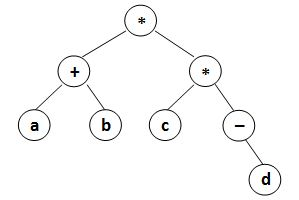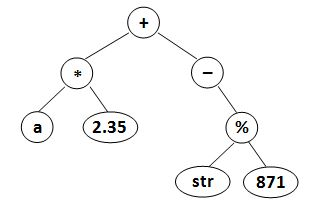Given a syntax tree (binary), you are supposed to output the corresponding infix expression, with parentheses reflecting the precedences of the operators.

## Input Specification:

Each input file contains one test case. For each case, the first line gives a positive integer N (≤ 20) which is the total number of nodes in the syntax tree. Then N lines follow, each gives the information of a node (the i-th line corresponds to the i-th node) in the format:

data left_child right_child
where data is a string of no more than 10 characters, left_child and right_child are the indices of this node's left and right children, respectively. The nodes are indexed from 1 to N. The NULL link is represented by −1. The figures 1 and 2 correspond to the samples 1 and 2, respectively.Figure 1Figure 2

## Output Specification:

For each case, print in a line the infix expression, with parentheses reflecting the precedences of the operators. Note that there must be no extra parentheses for the final expression, as is shown by the samples. There must be no space between any symbols.

8
* 8 7
a -1 -1
* 4 1
+ 2 5
b -1 -1
d -1 -1
- -1 6
c -1 -1

(a+b)(c(-d))
Sample Input 2:
8
2.35 -1 -1
* 6 1
- -1 4
% 7 8
+ 2 3
a -1 -1
str -1 -1
871 -1 -1

## Sample Output 2:

(a*2.35)+(-(str%871))

## 解答

#include<iostream>
#include<string>
#include<vector>

using namespace std;

struct node {
string data;
int left, right;
};
vector<node> nodes;

int root = -1;

string InOrder(int r)
{
if (nodes[r].left < 0 && nodes[r].right < 0)
return nodes[r].data;
else if (nodes[r].left < 0 && nodes[r].right > 0)
return "(" + nodes[r].data + InOrder(nodes[r].right) + ")";
else if (nodes[r].left > 0 && nodes[r].right > 0)
return "(" + InOrder(nodes[r].left) + nodes[r].data + InOrder(nodes[r].right) + ")";
}
int main()
{
int nodesN, sum1 = 0, sum2 = 0;
cin >> nodesN;
nodes.resize(nodesN + 1);
for (int i = 1; i <= nodesN; i++) {
cin >> nodes[i].data >> nodes[i].left >> nodes[i].right;
if (nodes[i].right > 0) sum1 += nodes[i].right;
if (nodes[i].left > 0) sum1 += nodes[i].left;
sum2 += i;
}
root = sum2 - sum1;
string ans = InOrder(root).c_str();
if (ans == '(') ans = ans.substr(1, ans.size() - 2);
printf("%s", ans.c_str());
return 0;
}